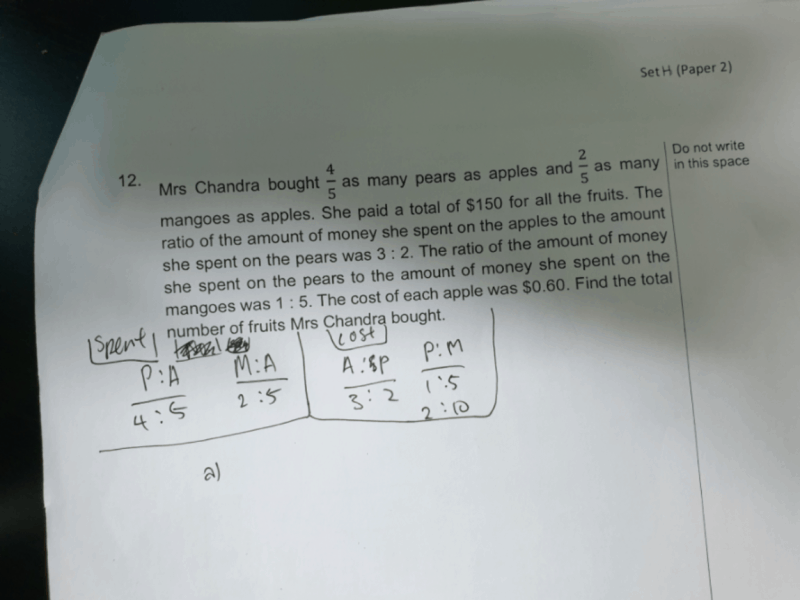# AnswerI used what you used for the ratios, but combined them together.

The question states that the total cost is \$150, which is 15 units since i added the numbers in the “cost” ratio. Then, I went to find 3 units, the cost of the apples. I then got \$30.

Since we know that the cost of one apple is \$0.60, we can do:\$30÷\$0.60=50.

Now, we need to find the number of pears and mangoes which is 40 and 20 respectively. With this, we add 40, 20, 50 together. The answer is 110 which is the total number of fruits.

0 Replies 0 Likes